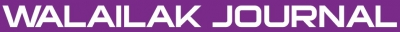### An Accurate Solution for the Steady Flow of Third-Grade Fluid in a Porous Half Space

Saeid ABBASBANDY, Hadi Roohani GHEHSAREH, Ishak HASHIM

#### Abstract

In this paper, a simple and efficient analysis technique, Hankel-Pade method, is proposed to find the solution of anonlinear ordinary differential equation that appears in the studyof the steady flow of the third-grade fluid in a porous halfspace. The numerical solutions  for some cases of the model'sparameters are obtained by using Hankel-Pade method. Theconvergence of the Hankel sequences is analyzed. Comparison toother available results for these problems reveals reliability andhigh accuracy of the proposed technique. Also the simpleanalytical expressions of the solutions of the governingnon-linear boundary-layer problems are developed as rationalapproximation solutions. The effectivity and convergence of therational approximation solutions are investigated by theillustrative graphs and tables.

PDF

#### References

KR Rajagopal. On the Boundary Conditions for the Fluid of the Differential Type. In: A Squire (ed.). Navier-Stokes Equations and Related Non-linear Problems. Plenum Press, New York, 1995, p. 273-8.

M Dehghan and R Salehi. Solution of a nonlinear time-delay model in biology via semi-analytical approaches. Comput. Phys. Commun. 2010; 181, 1255-65.

C Chun and R Sakthivel. Homotopy perturbation technique for solving two-point boundary value problems - comparison with other methods. Comput. Phys. Commun. 2010; 181, 1021-4.

S Liang and DJ Jeffrey. An effcient analytical approach for solving fourth order boundary value problems. Comput. Phys. Commun. 2009; 180, 2034-40.

FM Fernández, Q Ma and RH Tipping. Tight upper and lower bounds for energy eigenvalues of the Schrödinger equation. Phys. Rev. A. 1989; 39, 1605-9.

FM Fernández. Strong coupling expansion for anharmonic oscillators and perturbed Coulomb potentials. Phys. Lett. A. 1992; 166, 173-6.

FM Fernández and R Guardiola. Accurate eigenvalues and eigenfunctions for quantum-mechanical anharmonic oscillators. J. Phys. A. 1993; 26, 7169-80.

FM Fernández. Direct calculation of accurate Siegert eigenvalues. J. Phys. A: Math. Gen. 1995; 28, 4043-51.

FM Fernández. Alternative treatment of separable quantum-mechanical models: The hydrogen molecular ion. J. Chem. Phys. 1995; 103, 6581-5.

FM Fernández. Resonances for a perturbed Coulomb potential. Phys. Lett. A. 1995; 203, 275-8.

FM Fernández. Quantization condition for bound and quasibound states. J. Phys. A: Math. Gen. 1996; 29, 3167-77.

FM Fernández. Direct calculation of Stark resonances in hydrogen. Phys. Rev. A. 1996; 54, 1206-9.

FM Fernández. Tunnel resonances for one-dimensional barriers. Chem. Phys. Lett. 1997; 281, 337-42.

FM Fernández. On a perturbation treatment of a model for MHD viscous flow. Appl. Math. Comput. 2010; 217, 2307-10.

FM Fernández. Rational approximation to the Thomas-Fermi equations. Appl. Math. Comput. 2011; 217, 6433-6.

S Abbasbandy and T Hayat. Solution of the MHD Falkner-Skan flow by Hankel-Padé method. Phys. Lett. A. 2009; 373, 731-4.

B Boisseau, P Forgács and H Giacomini. An analytical approximation scheme to two-point boundary value problems of ordinary differential equations. J. Phys. A: Math. Theor. A. 2007; 40, F215-F222.

C Bervillier, B Boisseau and H Giacomini. Analytical approximation schemes for solving exact renormalization group equations in the local potential approximation. Nucl. Phys. B. 2008; 789, 525-51.

C Bervillier, B Boisseau and H Giacomini. Analytical approximation schemes for solving exact renormalization group equations. II Conformal mappings. Nucl. Phys. B. 2008; 801, 296-315.

T Hayat, F Shahzad and M Ayub. Analytical solution for the steady flow of the third-grade fluid in a porous half space. Appl. Math. Model. 2007; 31, 2424-32.

T Hayat, F Shahzad, M Ayub and S Asghar. Stokes’ first problem for a third-grade fluid in a porous half space. Comm. Nonlinear Sci. Numer. Simulat. 2008; 13, 1801-7.

F Ahmad. A simple analytical solution for the steady flow of the third-grade fluid in a porous half space. Comm. Nonlinear Sci. Numer. Simulat. 2009; 14, 2848-52.

SJ Liao. Beyond Perturbation: Introduction to the Homotopy Analysis Method. Chapman Hall/CRC Press, Boca Raton, London 2003.

S Abbasbandy and T Hayat. Solution of the MHD Falkner-Skan flow by homotopy analysis method. Comm. Nonlinear Sci. Numer. Simulat. 2009; 14, 3591-8.

TY Na. Computational Methods in Engineering Boundary Value Problems. Academic Press, New York, 1979.

FM Fernández. Comment on: “Series solution to the Thomas-Fermi equation”. Phys. Lett. A. 2008; 372, 5258-60.

K Mansour. Using Stokes expansion for natural convection inside a two-dimensional cavity. Fluid Dynam. Res. 1993; 12, 1-33.

### Refbacks

• There are currently no refbacks.Online ISSN: 2228-835X

http://wjst.wu.ac.th

Last updated: 20 June 2019